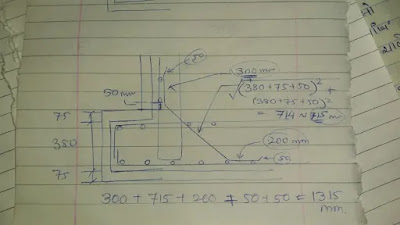Haunch Bar Cutting Length Calculation || Civil Experiences

Haunch bar Length calculation for estimation of reinforcements bar needs in the work is the most essential part because without estimation no idea of

Haunch bar length calculation

Haunch Bar Cutting Length Calculation for estimation of reinforcements bar needs in the work is the most essential part because without estimation you have no idea about how many quantities required for haunch bar cutting length in building project construction

Found out haunch bar length

Here I take an example of how to calculate haunch bar for a better understanding of how to calculate cutting length of haunch bar

The example gives you the flow of calculation methods because every site requires a different kind of design due to different kind of soil, environmental conditions, groundwater level, temperature etc

So here I considered 3 steps for Haunch Bar Cutting Length Calculation

Step consideration in Haunch Bar Cutting Length CalculationHaunch bar length calculation

Step 1: Diagonal length Haunch Bar

The diagonal length of the haunch bar

For diagonal calculation, we use Pythagoras theorem

Pythagoras theorem is diagonal length is equal to the square root of the sum of both side square sum

Like

AC^2 = AB^2 + BC^2

Where B has 90 angels

In our Design

Diagonal length = Square root ( square of ( 380 + 75 + 50) ) + ( square of ( 380 + 75 + 50 ) )

Diagonal length = 714 mm

Here we cal take 715 mm for easy simple calculation

Where

380+75+50 is the total vertical and also horizontal length in your case may be is different so be care full

Step 2: Supporting length of Haunch Bar

Now we adding value which given after diagonal length in Haunch Bar Cutting Length Calculation

Here additional value is 300 mm for the upper side and 200 mm lower side

Step 3: Hook length of Haunch Bar

The Third Step is adding hook length in Haunch Bar Cutting Length Calculation

Here is 50 mm for both ends

Final total length of haunch bar  = 50 + 300 + 715 + 200 + 50

Total length of haunch bar = 1315 mm

Note: is depend on your design requirement of the structure may be is some case is not provided when not provided then ignore it or assume its value zero

You may like these posts

1. To insert a code use <i rel="pre">code_here</i>
2. To insert a quote use <b rel="quote">your_qoute</b>
3. To insert a picture use <i rel="image">url_image_here</i>